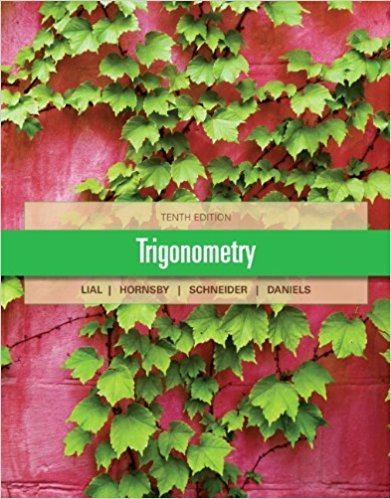×
×

# Solutions for Chapter 5.4: Sum and Difference Identities for Sine and Tangent## Full solutions for Trigonometry | 10th Edition

ISBN: 9780321671776Solutions for Chapter 5.4: Sum and Difference Identities for Sine and Tangent

Solutions for Chapter 5.4
4 5 0 353 Reviews
11
0
##### ISBN: 9780321671776

This textbook survival guide was created for the textbook: Trigonometry, edition: 10. Since 82 problems in chapter 5.4: Sum and Difference Identities for Sine and Tangent have been answered, more than 32661 students have viewed full step-by-step solutions from this chapter. Trigonometry was written by and is associated to the ISBN: 9780321671776. This expansive textbook survival guide covers the following chapters and their solutions. Chapter 5.4: Sum and Difference Identities for Sine and Tangent includes 82 full step-by-step solutions.

Key Math Terms and definitions covered in this textbook
• Augmented matrix [A b].

Ax = b is solvable when b is in the column space of A; then [A b] has the same rank as A. Elimination on [A b] keeps equations correct.

• Big formula for n by n determinants.

Det(A) is a sum of n! terms. For each term: Multiply one entry from each row and column of A: rows in order 1, ... , nand column order given by a permutation P. Each of the n! P 's has a + or - sign.

• Block matrix.

A matrix can be partitioned into matrix blocks, by cuts between rows and/or between columns. Block multiplication ofAB is allowed if the block shapes permit.

• Column picture of Ax = b.

The vector b becomes a combination of the columns of A. The system is solvable only when b is in the column space C (A).

• Complete solution x = x p + Xn to Ax = b.

(Particular x p) + (x n in nullspace).

• Eigenvalue A and eigenvector x.

Ax = AX with x#-O so det(A - AI) = o.

• Gauss-Jordan method.

Invert A by row operations on [A I] to reach [I A-I].

• Hermitian matrix A H = AT = A.

Complex analog a j i = aU of a symmetric matrix.

• Hessenberg matrix H.

Triangular matrix with one extra nonzero adjacent diagonal.

• Jordan form 1 = M- 1 AM.

If A has s independent eigenvectors, its "generalized" eigenvector matrix M gives 1 = diag(lt, ... , 1s). The block his Akh +Nk where Nk has 1 's on diagonall. Each block has one eigenvalue Ak and one eigenvector.

• Kirchhoff's Laws.

Current Law: net current (in minus out) is zero at each node. Voltage Law: Potential differences (voltage drops) add to zero around any closed loop.

• Left inverse A+.

If A has full column rank n, then A+ = (AT A)-I AT has A+ A = In.

• Multiplication Ax

= Xl (column 1) + ... + xn(column n) = combination of columns.

• Rank one matrix A = uvT f=. O.

Column and row spaces = lines cu and cv.

• Reflection matrix (Householder) Q = I -2uuT.

Unit vector u is reflected to Qu = -u. All x intheplanemirroruTx = o have Qx = x. Notice QT = Q-1 = Q.

• Schur complement S, D - C A -} B.

Appears in block elimination on [~ g ].

• Stiffness matrix

If x gives the movements of the nodes, K x gives the internal forces. K = ATe A where C has spring constants from Hooke's Law and Ax = stretching.

• Subspace S of V.

Any vector space inside V, including V and Z = {zero vector only}.

• Triangle inequality II u + v II < II u II + II v II.

For matrix norms II A + B II < II A II + II B II·

• Vector v in Rn.

Sequence of n real numbers v = (VI, ... , Vn) = point in Rn.

×